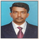1385 views
Hello Friends,

i found here a Keltner Channel Strategy. But this Strategy trades LONG and SHORT.

Could some code it to long also.

If a sell condtion is true, so no short trades is initiated - only sell se long trade to be flat.

is it possible?

Thank you so much for your help!

## KommentareSchopplMarcus
@SchopplMarcus, //@version=3
strategy("Keltner Channel Strategy", overlay=true)
source = close

useTrueRange = input(true)
length = input(20, minval=1)
mult = input(1.0)

ma = sma(source, length)
range = useTrueRange ? tr : high - low
rangema = sma(range, length)
upper = ma + rangema * mult
lower = ma - rangema * mult

crossUpper = crossover(source, upper)
crossLower = crossunder(source, lower)

bprice = 0.0
bprice := crossUpper ? high+syminfo.mintick : nz(bprice)

sprice = 0.0
sprice := crossLower ? low -syminfo.mintick : nz(sprice)

crossBcond = false
crossBcond := crossUpper ? true
: na(crossBcond) ? false : crossBcond

crossScond = false
crossScond := crossLower ? true
: na(crossScond) ? false : crossScond

cancelBcond = crossBcond and (source < ma or high >= bprice )
cancelScond = crossScond and (source > ma or low <= sprice )

if (cancelBcond)
strategy.cancel("KltChLE")

if (crossUpper)
strategy.entry("KltChLE", strategy.long, stop=bprice, comment="KltChLE")

//plot(strategy.equity, title="equity", color=red, linewidth=2, style=areabr)
Antworten